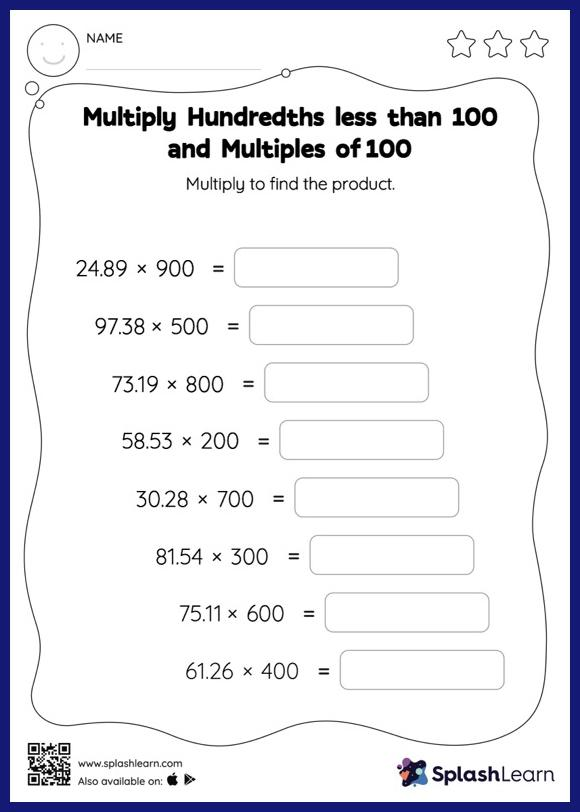# Multiply Hundredths less than 100 and Multiples of 100: Horizontal Multiplication Worksheet

Home > Multiply Hundredths less than 100 and Multiples of 100: Horizontal MultiplicationStudents demonstrate their problem-solving ability as they work on this multiply hundredths less than 100 and multiples of 100 worksheet. When students multiply a decimal by a number ending in zeros, they first ignore the decimal point, multiply the numbers, and then add the decimal point in the product as many places from the right as the number of decimal digits in the original number. In this worksheet, students practice solving problems written in the horizontal format. How numbers are laid out in a problem affects the method a student employs to solve it. Therefore to develop actual fluency and mastery of multiple strategies, students must practice different formats.